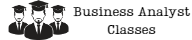Search

# The Benefits of Random Forests for Predictive Modeling

Random Forests is a widely used algorithm in machine learning, especially in predictive modeling. It is a type of decision tree algorithm that builds multiple decision trees and combines them to get more accurate and stable predictions. Random Forests can be used for both classification and regression tasks.In this article, we will discuss the benefits of Random Forests for predictive modeling. We will cover the following topics:

1. Introduction to Random Forests
3. Handling missing values
4. Feature importance
5. Outlier detection
6. Model interpretability
7. Conclusion

## Introduction to Random Forests

Random Forests is a popular algorithm that can handle large datasets with high dimensionality. It is a type of ensemble learning method that builds multiple decision trees and combines them to get more accurate and stable predictions. The basic idea behind Random Forests is to build a forest of decision trees, where each tree is built using a random sample of the training data and a random subset of the features. The final prediction is made by taking the average of the predictions of all the trees in the forest.

Random Forests can be used for classification and regression tasks. In classification tasks, the algorithm predicts the class of a new data point based on the majority class of the trees in the forest. In regression tasks, it predicts the value of a continuous target variable based on the average of the target variable of the trees in the forest.

Random Forests have several advantages over other machine learning algorithms. Some of the key advantages are:

### 1. High accuracy

Random Forests can achieve high accuracy in both classification and regression tasks. The combination of multiple decision trees reduces the variance and overfitting, which leads to more accurate predictions.

### 2. Robustness to noise and outliers

Random Forests are robust to noisy and outlier data points. The algorithm uses a random subset of the features and data points to build each tree, which reduces the impact of noisy and outlier data points on the final prediction.

### 3. Non-parametric

Random Forests are non-parametric, which means they do not make any assumptions about the distribution of the data. This makes them suitable for a wide range of data types and distributions.

### 4. Scalability

Random Forests can handle large datasets with high dimensionality. The algorithm can also be parallelized, which makes it scalable to even larger datasets.

### 5. Low bias

Random Forests have low bias, which means they can capture complex non-linear relationships between the features and the target variable.

## Handling missing values

One of the challenges in predictive modeling is handling missing values in the dataset. Random Forests have a built-in mechanism to handle missing values. When building each tree, the algorithm randomly selects a subset of the available features to split on. If a data point has a missing value for a feature, it is still included in the subset and the split is made based on the available features.

## Feature importance

Random Forests can also provide a measure of feature importance. The algorithm calculates the reduction in the impurity (Gini index or entropy) of the target variable when a feature is used to split the data. The features with the highest reduction in impurity are considered more important in predicting the target variable.

## Outlier detection

Random Forests can also be used for outlier detection. The algorithm can identify data points that are significantly different from the majority of the data points. This can be useful in detecting anomalies or fraud in the data.

## Model interpretability

One of the criticisms of machine learning algorithms is their lack of interpretability. Random Forests can provide some level of model interpretability by calculating the feature importance and visualizing the decision tree. This can help in understanding which features are important in predicting the target variable and how the algorithm is making the predictions.

## Conclusion

Random Forests is a powerful algorithm in predictive modeling that has several advantages over other machine learning algorithms. It can achieve high accuracy, handle missing values, provide a measure of feature importance, detect outliers, and provide some level of model interpretability. By understanding the benefits of Random Forests, data scientists can make more informed decisions when choosing an algorithm for their predictive modeling tasks.

### Try some of our classes

Enter your email and we’ll send you some samples of our favorite classes.

#### Course Categories

Quisque velit nisi, pretium ut lacinia in, elementum id enim.